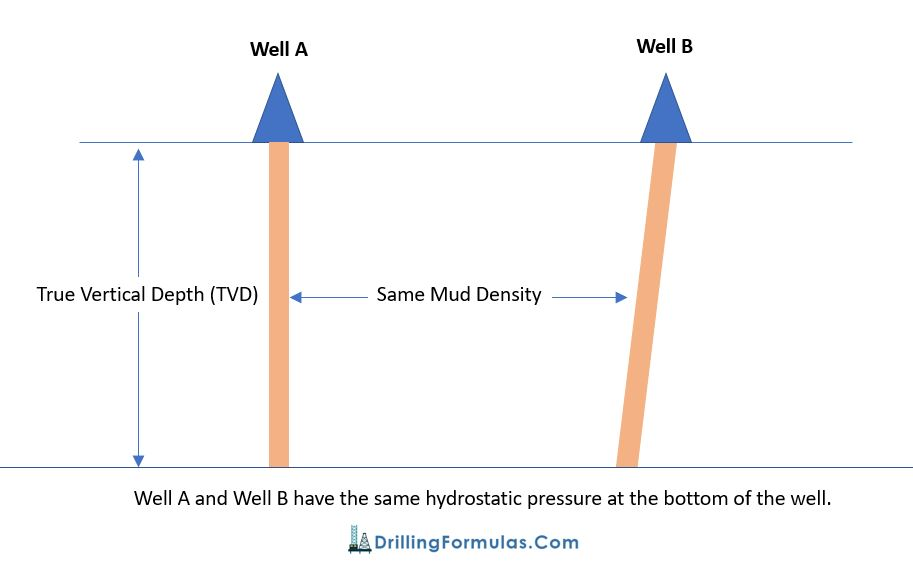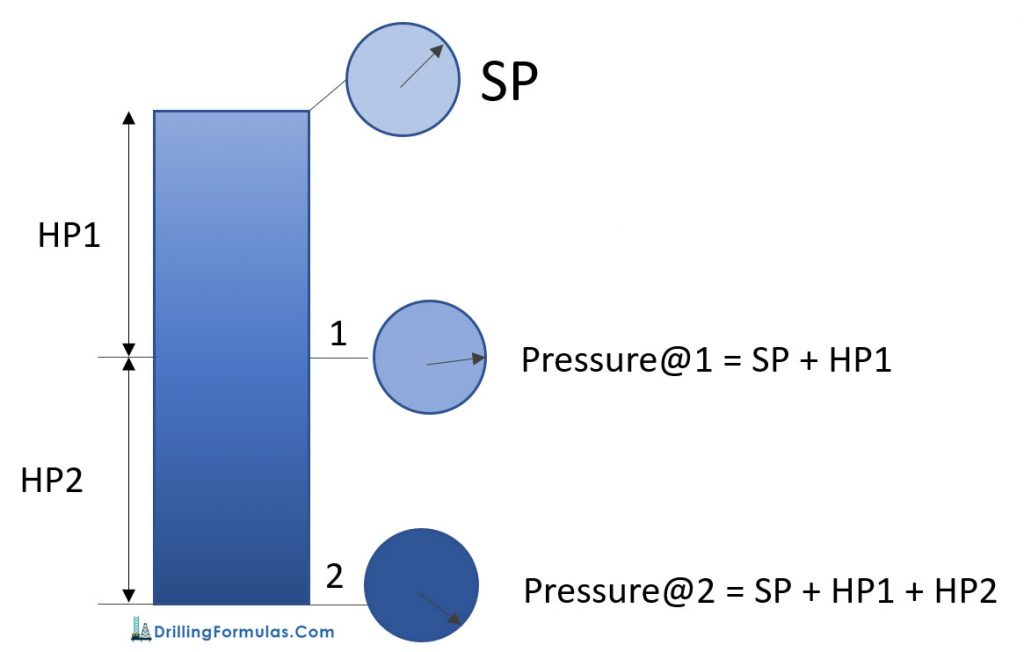# Understand Hydrostatic Pressure

In a well, any pressure created by a static column of fluid is called ‘Hydrostatic Pressure’ (HP); at any given True Vertical Depth (TVD). With ‘hydro’ representing water, which exerts pressure, ‘static’ means it has no movement. Any pressure developed by a column of fluid that isn’t moving, therefore, can be considered hydrostatic pressure; fluid in this sense can be either liquid or gas.

The relationship of hydrostatic pressure is shown in the equation below.

HP (Hydrostatic Pressure) = density x g (gravity acceleration) x h (True Vertical Depth, TVD)

In oilfield term, the formula above is modified so that people can use it easily. The formulas are as follows:

HP (Hydrostatic Pressure) = Constant x MW (Mud Weight or Mud Density)  x TVD (True Vertical Depth)

HP (psi)  = 0.052 x MW (ppg) x TVD (ft) ** Most frequent used in the oilfield **

HP (psi) = 0.007 x MW (pcf) x TVD (ft)

HP (kPa) = 0.00981 x MW (kg/m3) x TVD (m)

Depending on which unit is used for calculation, there are several conversion factors such as 0.052, 0.007, 0.00981 for instant as you can see from the equations above.

According to the equations above, Hydrostatic Pressure is not a function of hole geometry. Only mud weight and True Vertical Depth (TVD) affect on Hydrostatic Pressure. For example (a picture below); well A and well B have the same vertical depth. With the same mud density in hole, the bottom hole pressure due to hydrostatic pressure is the same. The only different between Well A and Well B is mud volume.This concept is basic and very important for many aspects such as well control, balance cementing, u-tube, etc.

## Pressure in a well

In a static condition

• Pressure at any depth = Hydrostatic Pressure (HP) + Surface Pressure (SP)
• Pressure between 2 points is HP between these points

The diagram below demonstrates the relationship of pressure in a well.

At point 1, Pressure@1 = Surface Pressure (SP) + Hydrostatic Pressure @ 1 (HP1)

At point 1, Pressure@2 = Surface Pressure (SP) + Hydrostatic Pressure@1 (HP1) + Hydrostatic Pressure@2 (HP2)Ref books:

Lapeyrouse, N.J., 2002. Formulas and calculations for drilling, production and workover, Boston: Gulf Professional publishing.

Bourgoyne, A.J.T., Chenevert , M.E. & Millheim, K.K., 1986. SPE Textbook Series, Volume 2: Applied Drilling Engineering, Society of Petroleum Engineers.

Mitchell, R.F., Miska, S. & Aadny, B.S., 2011. Fundamentals of drilling engineering, Richardson, TX: Society of Petroleum Engineers.

Share the joy
Tagged , . Bookmark the permalink.Working in the oil field and loving to share knowledge.

### 7 Responses to Understand Hydrostatic Pressure

1.Doug Hubbard says:

We do Horizontal Directional Boring with hole diameters upward to 54″ through which we pull a 36″ HDPE pipe.
The depths are geneally only 20-40 feet deep and the lenghts are upwards to 2000 lineal feet.
We are constantly in discussions that we need to be deeper to help prevent frac outs and potential groung heave issues with the Engineers reoresenting our customers.
I know that there are many variables that impct these issues, but it would be most helpful to be able to identify where the ground pressures vs hyrostatic indicate potential problems in soil conditions of sand, clay, or rock.
Can you help?
Thanks.

This site uses Akismet to reduce spam. Learn how your comment data is processed.